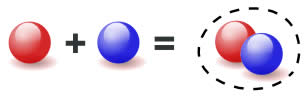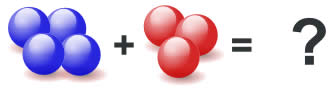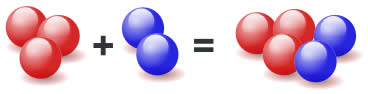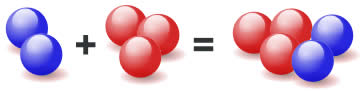... bringing two or more numbers (or things) together to make a new total.

 Here 1 ball is added to 1 ball to make 2 balls:Using Numerals it is: 1 + 1 = 2 And in words it is: "One plus one equals two"

### Example: When we add 2 and 3 we get 5.

We can write it like this:

2 + 3 = 5

## Try It

Write this down, with the answer, using numerals:You should get:   4 + 3 = 7

## Have a Play

Drag the numerals to the two blue boxes see how addition works:

## Swapping Places

Swapping the position of the numbers we are adding still gets the same result!

 3 + 2 = 5... also ... 2 + 3 = 5More Examples:

5 + 1 = 1 + 5 = 6

7 + 11 = 11 + 7 = 18

4 + 100 = 100 + 4 = 104

## PracticeYou get better at addition with practice, so we have:

## Tips and Tricks

We can also "look up" answers for simple addition using the Addition Table (but it is really best to learn to remember the answers).

+ 1 2 3 4 5 6 7
1 2 3 4 5 6 7 8
2 3 4 5 6 7 8 9
3 4 5 6 7 8 9 10
4 5 6 7 8 9 10 11

### Example: Find 3 + 5

• find the row starting with "3"

• move along to be under the column "5"

• and there is the number "8", so 3 + 5 = 8

## Larger Numbers: Use Addition in Columns

 To add larger numbers use Column Addition :## Names

Other names for Addition are Sum, Plus, Increase, Total